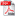# SOME ASPECTS REGARDING STRESS WAVE PROPAGATION IN TRONCONICAL SHORT BARS WITH VARIABLE ANGLE

Autor/autori: Nicolae ILIESCU, Vasile NĂSTĂSESCU, Costică ATANASIU

Abstract: In the first part of the paper an algorithm of calculus, using FEM, developed by the authors in a previous paper, is presented. This computational algorithm was applied in the study of stresses wave propagation produced by an axial impact in a tronconical short bar. Calculation methodology allowed determination of σr and σθ stresses distribution, produced by the wavefront in different cross sections of the bar. Calculation methodology developed was validated experimentally using three investigative techniques combined: dynamic photoelasticity, electroresistive strain gauge technique and laser-photomultiplier technique. In the second part of the paper this calculation methodology is applied to investigate the particularities involved in stresses distribution in the cross sections of short conical bars, with variable angle peak, subjected to an axial impact. The results of performed simulations are analyzed comparatively, settling influence of reflected waves on the stresses distribution in the cross sections of bars

Keywords: stress waves, photoelasticity, electrical stress measurement, finite element methodDOWNLOAD PDF